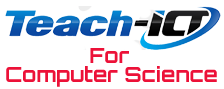#THE education site for computer science and ICT

This page is part of the old A451 specification - current syllabus here

## 1. Introduction

##### Specification point:

i) Convert positive denary whole numbers (0-255) into 8-bit binary numbers and vice versa

ii) Add two 8-bit binary integers and explain overflow errors which may occur

iii) Convert positive denary whole numbers (0-255) into 2-digit hexadecimal numbers and vice versa

iv) Convert between binary and hexadecimal equivalents of the same number

v) Explain the use of hexadecimal numbers to represent binary numbers